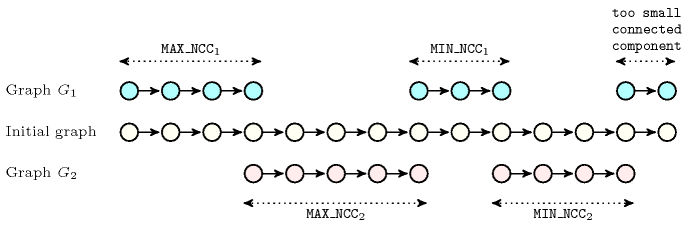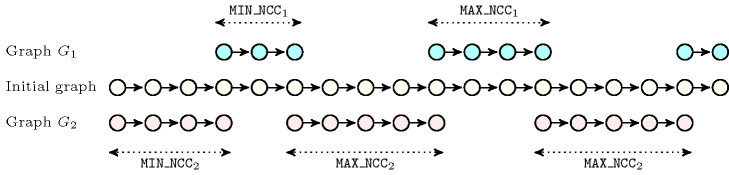### 4.3.4.9. five parameters/two final graphs

$\mathrm{𝐌𝐀𝐗}_{\mathrm{𝐍𝐂𝐂}}_{1}$, $\mathrm{𝐌𝐀𝐗}_{\mathrm{𝐍𝐂𝐂}}_{2}$, $\mathrm{𝐌𝐈𝐍}_{\mathrm{𝐍𝐂𝐂}}_{1}$, $\mathrm{𝐌𝐈𝐍}_{\mathrm{𝐍𝐂𝐂}}_{2}$, ${\mathrm{𝐍𝐂𝐂}}_{1}$

Proposition 151

$\begin{array}{cc}& \mathrm{𝚟𝚙𝚊𝚛𝚝𝚒𝚝𝚒𝚘𝚗}\wedge \mathrm{𝚌𝚘𝚗𝚜𝚎𝚌𝚞𝚝𝚒𝚟𝚎}_\mathrm{𝚕𝚘𝚘𝚙𝚜}_\mathrm{𝚊𝚛𝚎}_\mathrm{𝚌𝚘𝚗𝚗𝚎𝚌𝚝𝚎𝚍}:\hfill \\ & \mathrm{𝐌𝐈𝐍}_{\mathrm{𝐍𝐂𝐂}}_{1}·max\left(0,{\mathrm{𝐍𝐂𝐂}}_{1}-1\right)+\mathrm{𝐌𝐀𝐗}_{\mathrm{𝐍𝐂𝐂}}_{1}+\hfill \\ & \mathrm{𝐌𝐈𝐍}_{\mathrm{𝐍𝐂𝐂}}_{2}·max\left(0,{\mathrm{𝐍𝐂𝐂}}_{1}-2\right)+\mathrm{𝐌𝐀𝐗}_{\mathrm{𝐍𝐂𝐂}}_{2}\le {\mathrm{𝐍𝐕𝐄𝐑𝐓𝐄𝐗}}_{\mathrm{𝙸𝙽𝙸𝚃𝙸𝙰𝙻}}\hfill \end{array}$

Proof 149 The left-hand side of 151 corresponds to the minimum number of vertices of the two final graphs provided that we build the smallest possible connected components.

Proposition 152

$\begin{array}{cc}& \mathrm{𝚟𝚙𝚊𝚛𝚝𝚒𝚝𝚒𝚘𝚗}\wedge \mathrm{𝚌𝚘𝚗𝚜𝚎𝚌𝚞𝚝𝚒𝚟𝚎}_\mathrm{𝚕𝚘𝚘𝚙𝚜}_\mathrm{𝚊𝚛𝚎}_\mathrm{𝚌𝚘𝚗𝚗𝚎𝚌𝚝𝚎𝚍}:\hfill \\ & {\mathrm{𝐍𝐂𝐂}}_{1}\le \left(\overline{\mathrm{𝐌𝐀𝐗}_{\mathrm{𝐍𝐂𝐂}}_{1}}>0\right)+⌊\frac{\alpha }{\beta }⌋+\left(\alpha \mathrm{mod}\beta \ge max\left(1,\underline{\mathrm{𝐌𝐈𝐍}_{\mathrm{𝐍𝐂𝐂}}_{1}}\right)\right)\hfill \\ & \left\{\begin{array}{c}•\alpha =max\left(0,{\mathrm{𝐍𝐕𝐄𝐑𝐓𝐄𝐗}}_{\mathrm{𝙸𝙽𝙸𝚃𝙸𝙰𝙻}}-max\left(1,\underline{\mathrm{𝐌𝐀𝐗}_{\mathrm{𝐍𝐂𝐂}}_{1}}\right)-max\left(1,\underline{\mathrm{𝐌𝐀𝐗}_{\mathrm{𝐍𝐂𝐂}}_{2}}\right)\right),\hfill \\ •\beta =max\left(1,\underline{\mathrm{𝐌𝐈𝐍}_{\mathrm{𝐍𝐂𝐂}}_{1}}\right)+max\left(1,\underline{\mathrm{𝐌𝐈𝐍}_{\mathrm{𝐍𝐂𝐂}}_{2}}\right).\hfill \end{array}\right\\hfill \end{array}$

Proof 150 The maximum number of connected components is achieved by building non-empty groups as small as possible, except for two groups of respective size $max\left(1,\underline{\mathrm{𝐌𝐀𝐗}_{\mathrm{𝐍𝐂𝐂}}_{1}}\right)$ and $max\left(1,\underline{\mathrm{𝐌𝐀𝐗}_{\mathrm{𝐍𝐂𝐂}}_{2}}\right)$, which have to be built.

Proposition 153

$\begin{array}{cc}& \mathrm{𝚟𝚙𝚊𝚛𝚝𝚒𝚝𝚒𝚘𝚗}\wedge \mathrm{𝚌𝚘𝚗𝚜𝚎𝚌𝚞𝚝𝚒𝚟𝚎}_\mathrm{𝚕𝚘𝚘𝚙𝚜}_\mathrm{𝚊𝚛𝚎}_\mathrm{𝚌𝚘𝚗𝚗𝚎𝚌𝚝𝚎𝚍}:\hfill \\ & \mathrm{𝐌𝐀𝐗}_{\mathrm{𝐍𝐂𝐂}}_{1}·max\left(0,{\mathrm{𝐍𝐂𝐂}}_{1}-1\right)+\mathrm{𝐌𝐈𝐍}_{\mathrm{𝐍𝐂𝐂}}_{1}+\hfill \\ & \mathrm{𝐌𝐀𝐗}_{\mathrm{𝐍𝐂𝐂}}_{2}·{\mathrm{𝐍𝐂𝐂}}_{1}+\mathrm{𝐌𝐈𝐍}_{\mathrm{𝐍𝐂𝐂}}_{2}\ge {\mathrm{𝐍𝐕𝐄𝐑𝐓𝐄𝐗}}_{\mathrm{𝙸𝙽𝙸𝚃𝙸𝙰𝙻}}\hfill \end{array}$

Proof 151 The left-hand side of 153 corresponds to the maximum number of vertices of the two final graphs provided that we build the largest possible connected components.

Proposition 154

$\begin{array}{cc}& \mathrm{𝚟𝚙𝚊𝚛𝚝𝚒𝚝𝚒𝚘𝚗}\wedge \mathrm{𝚌𝚘𝚗𝚜𝚎𝚌𝚞𝚝𝚒𝚟𝚎}_\mathrm{𝚕𝚘𝚘𝚙𝚜}_\mathrm{𝚊𝚛𝚎}_\mathrm{𝚌𝚘𝚗𝚗𝚎𝚌𝚝𝚎𝚍}:\hfill \\ & {\mathrm{𝐍𝐂𝐂}}_{1}\ge \left(\overline{\mathrm{𝐌𝐀𝐗}_{\mathrm{𝐍𝐂𝐂}}_{2}}<{\mathrm{𝐍𝐕𝐄𝐑𝐓𝐄𝐗}}_{\mathrm{𝙸𝙽𝙸𝚃𝙸𝙰𝙻}}\right)+⌊\frac{\alpha }{\beta }⌋+\left(\alpha \mathrm{mod}\beta >\overline{\mathrm{𝐌𝐀𝐗}_{\mathrm{𝐍𝐂𝐂}}_{2}}\right)\hfill \\ & \left\{\begin{array}{c}•\alpha =max\left(0,{\mathrm{𝐍𝐕𝐄𝐑𝐓𝐄𝐗}}_{\mathrm{𝙸𝙽𝙸𝚃𝙸𝙰𝙻}}-\overline{\mathrm{𝐌𝐈𝐍}_{\mathrm{𝐍𝐂𝐂}}_{1}}-\overline{\mathrm{𝐌𝐈𝐍}_{\mathrm{𝐍𝐂𝐂}}_{2}},\hfill \\ •\beta =max\left(1,\overline{\mathrm{𝐌𝐀𝐗}_{\mathrm{𝐍𝐂𝐂}}_{1}}\right)+max\left(1,\overline{\mathrm{𝐌𝐀𝐗}_{\mathrm{𝐍𝐂𝐂}}_{2}}\right).\hfill \end{array}\right\\hfill \end{array}$

Proof 152 The minimum number of connected components is achieved by taking the groups as large as possible except for two groups of respective size $\overline{\mathrm{𝐌𝐈𝐍}_{\mathrm{𝐍𝐂𝐂}}_{2}}$ and $\overline{\mathrm{𝐌𝐈𝐍}_{\mathrm{𝐍𝐂𝐂}}_{1}}$, which have to be built.

Proposition 155

$\begin{array}{cc}& \mathrm{𝚟𝚙𝚊𝚛𝚝𝚒𝚝𝚒𝚘𝚗}\wedge \mathrm{𝚌𝚘𝚗𝚜𝚎𝚌𝚞𝚝𝚒𝚟𝚎}_\mathrm{𝚕𝚘𝚘𝚙𝚜}_\mathrm{𝚊𝚛𝚎}_\mathrm{𝚌𝚘𝚗𝚗𝚎𝚌𝚝𝚎𝚍}:\hfill \\ & \mathrm{𝐌𝐀𝐗}_{\mathrm{𝐍𝐂𝐂}}_{2}\le max\left(\mathrm{𝐌𝐈𝐍}_{\mathrm{𝐍𝐂𝐂}}_{2},{\mathrm{𝐍𝐕𝐄𝐑𝐓𝐄𝐗}}_{\mathrm{𝙸𝙽𝙸𝚃𝙸𝙰𝙻}}-\alpha \right),\mathrm{𝚠𝚒𝚝𝚑}:\hfill \\ & •\alpha =\mathrm{𝐌𝐈𝐍}_{\mathrm{𝐍𝐂𝐂}}_{1}·max\left(0,{\mathrm{𝐍𝐂𝐂}}_{1}-1\right)+\mathrm{𝐌𝐀𝐗}_{\mathrm{𝐍𝐂𝐂}}_{1}+\hfill \\ & \mathrm{𝐌𝐈𝐍}_{\mathrm{𝐍𝐂𝐂}}_{2}+\mathrm{𝐌𝐈𝐍}_{\mathrm{𝐍𝐂𝐂}}_{2}·max\left(0,{\mathrm{𝐍𝐂𝐂}}_{1}-3\right)\hfill \end{array}$

Proof 153 If ${\mathrm{𝐍𝐂𝐂}}_{1}\le 1$ we have that $\mathrm{𝐌𝐀𝐗}_{\mathrm{𝐍𝐂𝐂}}_{2}\le \mathrm{𝐌𝐈𝐍}_{\mathrm{𝐍𝐂𝐂}}_{2}$. Otherwise, when ${\mathrm{𝐍𝐂𝐂}}_{1}>1$, we have that $\mathrm{𝐌𝐈𝐍}_{\mathrm{𝐍𝐂𝐂}}_{1}·max\left(0,{\mathrm{𝐍𝐂𝐂}}_{1}-1\right)+\mathrm{𝐌𝐀𝐗}_{\mathrm{𝐍𝐂𝐂}}_{1}+\mathrm{𝐌𝐈𝐍}_{\mathrm{𝐍𝐂𝐂}}_{2}+\mathrm{𝐌𝐀𝐗}_{\mathrm{𝐍𝐂𝐂}}_{2}+\mathrm{𝐌𝐈𝐍}_{\mathrm{𝐍𝐂𝐂}}_{2}·max\left(0,{\mathrm{𝐍𝐂𝐂}}_{1}-3\right)\le {\mathrm{𝐍𝐕𝐄𝐑𝐓𝐄𝐗}}_{\mathrm{𝙸𝙽𝙸𝚃𝙸𝙰𝙻}}$. ${\mathrm{𝐍𝐂𝐂}}_{1}-3$ comes from the fact that we build the minimum number of connected components in the second final graph (i.e., ${\mathrm{𝐍𝐂𝐂}}_{1}-1$ connected components) and that we have already built two connected components of respective size $\mathrm{𝐌𝐈𝐍}_{\mathrm{𝐍𝐂𝐂}}_{2}$ and $\mathrm{𝐌𝐀𝐗}_{\mathrm{𝐍𝐂𝐂}}_{2}$. By isolating $\mathrm{𝐌𝐀𝐗}_{\mathrm{𝐍𝐂𝐂}}_{2}$ in the previous expression and by grouping the two inequalities the result follows.

Proposition 156

$\begin{array}{cc}& \mathrm{𝚊𝚙𝚊𝚛𝚝𝚒𝚝𝚒𝚘𝚗}\wedge \mathrm{𝐚𝐫𝐜}_\mathrm{𝐠𝐞𝐧}=\mathrm{𝑃𝐴𝑇𝐻}\wedge \mathrm{𝐌𝐈𝐍}_{\mathrm{𝐍𝐂𝐂}}_{1}>1\wedge \mathrm{𝐌𝐈𝐍}_{\mathrm{𝐍𝐂𝐂}}_{2}>1:\hfill \\ & {\mathrm{𝐍𝐂𝐂}}_{1}\le \left(\mathrm{𝐌𝐀𝐗}_{\mathrm{𝐍𝐂𝐂}}_{1}>0\right)+⌊\frac{\alpha }{\beta }⌋+\left(\left(\alpha \mathrm{mod}\beta \right)+1\ge \mathrm{𝐌𝐈𝐍}_{\mathrm{𝐍𝐂𝐂}}_{1}\right),\mathrm{𝚠𝚒𝚝𝚑}:\hfill \\ & \left\{\begin{array}{c}•\alpha =max\left(0,{\mathrm{𝐍𝐕𝐄𝐑𝐓𝐄𝐗}}_{\mathrm{𝙸𝙽𝙸𝚃𝙸𝙰𝙻}}-\mathrm{𝐌𝐀𝐗}_{\mathrm{𝐍𝐂𝐂}}_{1}-\mathrm{𝐌𝐀𝐗}_{\mathrm{𝐍𝐂𝐂}}_{2}+1\right),\hfill \\ •\beta =\mathrm{𝐌𝐈𝐍}_{\mathrm{𝐍𝐂𝐂}}_{1}+\mathrm{𝐌𝐈𝐍}_{\mathrm{𝐍𝐂𝐂}}_{2}-2.\hfill \end{array}\right\\hfill \end{array}$

##### Figure 4.3.9. Illustration of Proposition 156. Configuration achieving the maximum number of connected components for ${G}_{1}$ according to the size of the smallest and largest connected components of ${G}_{1}$ and ${G}_{2}$ and to an initial number of vertices ($\mathrm{𝙼𝙰𝚇}_{\mathrm{𝙽𝙲𝙲}}_{1}=4,\mathrm{𝙼𝙰𝚇}_{\mathrm{𝙽𝙲𝙲}}_{2}=5,\mathrm{𝙼𝙸𝙽}_{\mathrm{𝙽𝙲𝙲}}_{1}=3,\mathrm{𝙼𝙸𝙽}_{\mathrm{𝙽𝙲𝙲}}_{2}=4,{\mathrm{𝙽𝚅𝙴𝚁𝚃𝙴𝚇}}_{\mathrm{𝙸𝙽𝙸𝚃𝙸𝙰𝙻}}=14,\alpha =max\left(0,14-4-5+1\right)=6,\beta =max\left(2,3+4-2\right)=5,{\mathrm{𝙽𝙲𝙲}}_{1}=\left(4>0\right)+⌊\frac{6}{5}⌋+\left(\left(\left(6\mathrm{mod}5\right)+1\right)\ge 3\right)=2$); since the two rightmost vertices of graph ${G}_{1}$ correspond to a too small connected component, they will have to be dispatched in the other connected components of graph ${G}_{1}$.Proof 154 The maximum number of connected components of ${G}_{1}$ is achieved by:

• Building a first connected component of ${G}_{1}$ involving $\mathrm{𝐌𝐀𝐗}_{\mathrm{𝐍𝐂𝐂}}_{1}$ vertices,

• Building a first connected component of ${G}_{2}$ involving $\mathrm{𝐌𝐀𝐗}_{\mathrm{𝐍𝐂𝐂}}_{2}$ vertices,

• Building alternatively a connected component of ${G}_{1}$ and a connected component of ${G}_{2}$ involving respectively $\mathrm{𝐌𝐈𝐍}_{\mathrm{𝐍𝐂𝐂}}_{1}$ and $\mathrm{𝐌𝐈𝐍}_{\mathrm{𝐍𝐂𝐂}}_{2}$ vertices,

• Finally, if this is possible, building a connected component of ${G}_{1}$ involving $\mathrm{𝐌𝐈𝐍}_{\mathrm{𝐍𝐂𝐂}}_{1}$ vertices.

Proposition 157

$\begin{array}{cc}& \mathrm{𝚊𝚙𝚊𝚛𝚝𝚒𝚝𝚒𝚘𝚗}\wedge \mathrm{𝐚𝐫𝐜}_\mathrm{𝐠𝐞𝐧}=\mathrm{𝑃𝐴𝑇𝐻}\wedge \mathrm{𝐌𝐈𝐍}_{\mathrm{𝐍𝐂𝐂}}_{1}>1\wedge \mathrm{𝐌𝐈𝐍}_{\mathrm{𝐍𝐂𝐂}}_{2}>1:\hfill \\ & {\mathrm{𝐍𝐂𝐂}}_{1}\ge \left(\mathrm{𝐌𝐈𝐍}_{\mathrm{𝐍𝐂𝐂}}_{1}>0\right)+⌊\frac{\alpha }{\beta }⌋+\left(\left(\alpha \mathrm{mod}\beta \right)+1>\mathrm{𝐌𝐀𝐗}_{\mathrm{𝐍𝐂𝐂}}_{2}\right),\mathrm{𝚠𝚒𝚝𝚑}:\hfill \\ & \left\{\begin{array}{c}•\alpha =max\left(0,{\mathrm{𝐍𝐕𝐄𝐑𝐓𝐄𝐗}}_{\mathrm{𝙸𝙽𝙸𝚃𝙸𝙰𝙻}}-\mathrm{𝐌𝐈𝐍}_{\mathrm{𝐍𝐂𝐂}}_{1}-\mathrm{𝐌𝐈𝐍}_{\mathrm{𝐍𝐂𝐂}}_{2}+1\right),\hfill \\ •\beta =\mathrm{𝐌𝐀𝐗}_{\mathrm{𝐍𝐂𝐂}}_{1}+\mathrm{𝐌𝐀𝐗}_{\mathrm{𝐍𝐂𝐂}}_{2}-2.\hfill \end{array}\right\\hfill \end{array}$

##### Figure 4.3.10. Illustration of Proposition 157. Configuration achieving the minimum number of connected components for ${G}_{1}$ according to the size of the smallest and largest connected components of ${G}_{1}$ and ${G}_{2}$ and to an initial number of vertices ($\mathrm{𝙼𝙰𝚇}_{\mathrm{𝙽𝙲𝙲}}_{1}=4,\mathrm{𝙼𝙰𝚇}_{\mathrm{𝙽𝙲𝙲}}_{2}=5,\mathrm{𝙼𝙸𝙽}_{\mathrm{𝙽𝙲𝙲}}_{1}=3,\mathrm{𝙼𝙸𝙽}_{\mathrm{𝙽𝙲𝙲}}_{2}=4,{\mathrm{𝙽𝚅𝙴𝚁𝚃𝙴𝚇}}_{\mathrm{𝙸𝙽𝙸𝚃𝙸𝙰𝙻}}=18,\alpha =max\left(0,18-3-4+1\right)=12,\beta =max\left(2,4+5-2\right)=7,{\mathrm{𝙽𝙲𝙲}}_{1}=\left(3>0\right)+⌊\frac{12}{7}⌋+\left(\left(\left(12\mathrm{mod}7\right)+1\right)>5\right)=3$)Proof 155 The minimum number of connected components of ${G}_{1}$ is achieved by:

• Building a first connected component of ${G}_{2}$ involving $\mathrm{𝐌𝐈𝐍}_{\mathrm{𝐍𝐂𝐂}}_{2}$ vertices,

• Building a first connected component of ${G}_{1}$ involving $\mathrm{𝐌𝐈𝐍}_{\mathrm{𝐍𝐂𝐂}}_{1}$ vertices,

• Building alternatively a connected component of ${G}_{2}$ and a connected component of ${G}_{1}$ involving respectively $\mathrm{𝐌𝐀𝐗}_{\mathrm{𝐍𝐂𝐂}}_{2}$ and $\mathrm{𝐌𝐀𝐗}_{\mathrm{𝐍𝐂𝐂}}_{1}$ vertices,

• Finally, if this is possible, building a connected component of ${G}_{2}$ involving $\mathrm{𝐌𝐀𝐗}_{\mathrm{𝐍𝐂𝐂}}_{2}$ vertices and a connected component of ${G}_{1}$ with the remaining vertices. Note that these remaining vertices cannot be incorporated in the connected components previously built.

$\mathrm{𝐌𝐀𝐗}_{\mathrm{𝐍𝐂𝐂}}_{1}$, $\mathrm{𝐌𝐀𝐗}_{\mathrm{𝐍𝐂𝐂}}_{2}$, $\mathrm{𝐌𝐈𝐍}_{\mathrm{𝐍𝐂𝐂}}_{1}$, $\mathrm{𝐌𝐈𝐍}_{\mathrm{𝐍𝐂𝐂}}_{2}$, ${\mathrm{𝐍𝐂𝐂}}_{2}$

Proposition 158

$\begin{array}{cc}& \mathrm{𝚟𝚙𝚊𝚛𝚝𝚒𝚝𝚒𝚘𝚗}\wedge \mathrm{𝚌𝚘𝚗𝚜𝚎𝚌𝚞𝚝𝚒𝚟𝚎}_\mathrm{𝚕𝚘𝚘𝚙𝚜}_\mathrm{𝚊𝚛𝚎}_\mathrm{𝚌𝚘𝚗𝚗𝚎𝚌𝚝𝚎𝚍}:\hfill \\ & \mathrm{𝐌𝐈𝐍}_{\mathrm{𝐍𝐂𝐂}}_{2}·max\left(0,{\mathrm{𝐍𝐂𝐂}}_{2}-1\right)+\mathrm{𝐌𝐀𝐗}_{\mathrm{𝐍𝐂𝐂}}_{2}+\hfill \\ & \mathrm{𝐌𝐈𝐍}_{\mathrm{𝐍𝐂𝐂}}_{1}·max\left(0,{\mathrm{𝐍𝐂𝐂}}_{2}-2\right)+\mathrm{𝐌𝐀𝐗}_{\mathrm{𝐍𝐂𝐂}}_{1}\le {\mathrm{𝐍𝐕𝐄𝐑𝐓𝐄𝐗}}_{\mathrm{𝙸𝙽𝙸𝚃𝙸𝙰𝙻}}\hfill \end{array}$

Proof 156 Similar to Proposition 151.

Proposition 159

$\begin{array}{cc}& \mathrm{𝚟𝚙𝚊𝚛𝚝𝚒𝚝𝚒𝚘𝚗}\wedge \mathrm{𝚌𝚘𝚗𝚜𝚎𝚌𝚞𝚝𝚒𝚟𝚎}_\mathrm{𝚕𝚘𝚘𝚙𝚜}_\mathrm{𝚊𝚛𝚎}_\mathrm{𝚌𝚘𝚗𝚗𝚎𝚌𝚝𝚎𝚍}:\hfill \\ & {\mathrm{𝐍𝐂𝐂}}_{2}\le \left(\overline{\mathrm{𝐌𝐀𝐗}_{\mathrm{𝐍𝐂𝐂}}_{2}}>0\right)+⌊\frac{\alpha }{\beta }⌋+\left(\alpha \mathrm{mod}\beta \ge max\left(1,\underline{\mathrm{𝐌𝐈𝐍}_{\mathrm{𝐍𝐂𝐂}}_{2}}\right)\right)\hfill \\ & \left\{\begin{array}{c}•\alpha =max\left(0,{\mathrm{𝐍𝐕𝐄𝐑𝐓𝐄𝐗}}_{\mathrm{𝙸𝙽𝙸𝚃𝙸𝙰𝙻}}-max\left(1,\underline{\mathrm{𝐌𝐀𝐗}_{\mathrm{𝐍𝐂𝐂}}_{2}}\right)-max\left(1,\underline{\mathrm{𝐌𝐀𝐗}_{\mathrm{𝐍𝐂𝐂}}_{1}}\right)\right),\hfill \\ •\beta =max\left(1,\underline{\mathrm{𝐌𝐈𝐍}_{\mathrm{𝐍𝐂𝐂}}_{2}}\right)+max\left(1,\underline{\mathrm{𝐌𝐈𝐍}_{\mathrm{𝐍𝐂𝐂}}_{1}}\right).\hfill \end{array}\right\\hfill \end{array}$

Proof 157 Similar to Proposition 152.

Proposition 160

$\begin{array}{cc}& \mathrm{𝚟𝚙𝚊𝚛𝚝𝚒𝚝𝚒𝚘𝚗}\wedge \mathrm{𝚌𝚘𝚗𝚜𝚎𝚌𝚞𝚝𝚒𝚟𝚎}_\mathrm{𝚕𝚘𝚘𝚙𝚜}_\mathrm{𝚊𝚛𝚎}_\mathrm{𝚌𝚘𝚗𝚗𝚎𝚌𝚝𝚎𝚍}:\hfill \\ & \mathrm{𝐌𝐀𝐗}_{\mathrm{𝐍𝐂𝐂}}_{2}·max\left(0,{\mathrm{𝐍𝐂𝐂}}_{2}-1\right)+\mathrm{𝐌𝐈𝐍}_{\mathrm{𝐍𝐂𝐂}}_{2}+\hfill \\ & \mathrm{𝐌𝐀𝐗}_{\mathrm{𝐍𝐂𝐂}}_{1}·{\mathrm{𝐍𝐂𝐂}}_{2}+\mathrm{𝐌𝐈𝐍}_{\mathrm{𝐍𝐂𝐂}}_{1}\ge {\mathrm{𝐍𝐕𝐄𝐑𝐓𝐄𝐗}}_{\mathrm{𝙸𝙽𝙸𝚃𝙸𝙰𝙻}}\hfill \end{array}$

Proof 158 Similar to Proposition 153.

Proposition 161

$\begin{array}{cc}& \mathrm{𝚟𝚙𝚊𝚛𝚝𝚒𝚝𝚒𝚘𝚗}\wedge \mathrm{𝚌𝚘𝚗𝚜𝚎𝚌𝚞𝚝𝚒𝚟𝚎}_\mathrm{𝚕𝚘𝚘𝚙𝚜}_\mathrm{𝚊𝚛𝚎}_\mathrm{𝚌𝚘𝚗𝚗𝚎𝚌𝚝𝚎𝚍}:\hfill \\ & {\mathrm{𝐍𝐂𝐂}}_{2}\ge \left(\overline{\mathrm{𝐌𝐀𝐗}_{\mathrm{𝐍𝐂𝐂}}_{1}}<{\mathrm{𝐍𝐕𝐄𝐑𝐓𝐄𝐗}}_{\mathrm{𝙸𝙽𝙸𝚃𝙸𝙰𝙻}}\right)+⌊\frac{\alpha }{\beta }⌋+\left(\alpha \mathrm{mod}\beta >\overline{\mathrm{𝐌𝐀𝐗}_{\mathrm{𝐍𝐂𝐂}}_{1}}\right)\hfill \\ & \left\{\begin{array}{c}•\alpha =max\left(0,{\mathrm{𝐍𝐕𝐄𝐑𝐓𝐄𝐗}}_{\mathrm{𝙸𝙽𝙸𝚃𝙸𝙰𝙻}}-\overline{\mathrm{𝐌𝐈𝐍}_{\mathrm{𝐍𝐂𝐂}}_{2}}-\overline{\mathrm{𝐌𝐈𝐍}_{\mathrm{𝐍𝐂𝐂}}_{1}},\hfill \\ •\beta =max\left(1,\overline{\mathrm{𝐌𝐀𝐗}_{\mathrm{𝐍𝐂𝐂}}_{2}}\right)+max\left(1,\overline{\mathrm{𝐌𝐀𝐗}_{\mathrm{𝐍𝐂𝐂}}_{1}}\right).\hfill \end{array}\right\\hfill \end{array}$

Proof 159 Similar to Proposition 154.

Proposition 162

$\begin{array}{cc}& \mathrm{𝚟𝚙𝚊𝚛𝚝𝚒𝚝𝚒𝚘𝚗}\wedge \mathrm{𝚌𝚘𝚗𝚜𝚎𝚌𝚞𝚝𝚒𝚟𝚎}_\mathrm{𝚕𝚘𝚘𝚙𝚜}_\mathrm{𝚊𝚛𝚎}_\mathrm{𝚌𝚘𝚗𝚗𝚎𝚌𝚝𝚎𝚍}:\hfill \\ & \mathrm{𝐌𝐀𝐗}_{\mathrm{𝐍𝐂𝐂}}_{1}\le max\left(\mathrm{𝐌𝐈𝐍}_{\mathrm{𝐍𝐂𝐂}}_{1},{\mathrm{𝐍𝐕𝐄𝐑𝐓𝐄𝐗}}_{\mathrm{𝙸𝙽𝙸𝚃𝙸𝙰𝙻}}-\alpha \right),\mathrm{𝚠𝚒𝚝𝚑}:\hfill \\ & •\alpha =\mathrm{𝐌𝐈𝐍}_{\mathrm{𝐍𝐂𝐂}}_{2}·max\left(0,{\mathrm{𝐍𝐂𝐂}}_{2}-1\right)+\mathrm{𝐌𝐀𝐗}_{\mathrm{𝐍𝐂𝐂}}_{2}+\hfill \\ & \mathrm{𝐌𝐈𝐍}_{\mathrm{𝐍𝐂𝐂}}_{1}+\mathrm{𝐌𝐈𝐍}_{\mathrm{𝐍𝐂𝐂}}_{1}·max\left(0,{\mathrm{𝐍𝐂𝐂}}_{2}-3\right)\hfill \end{array}$

Proof 160 Similar to Proposition 155.

Proposition 163

$\begin{array}{cc}& \mathrm{𝚊𝚙𝚊𝚛𝚝𝚒𝚝𝚒𝚘𝚗}\wedge \mathrm{𝐚𝐫𝐜}_\mathrm{𝐠𝐞𝐧}=\mathrm{𝑃𝐴𝑇𝐻}\wedge \mathrm{𝐌𝐈𝐍}_{\mathrm{𝐍𝐂𝐂}}_{1}>1\wedge \mathrm{𝐌𝐈𝐍}_{\mathrm{𝐍𝐂𝐂}}_{2}>1:\hfill \\ & {\mathrm{𝐍𝐂𝐂}}_{2}\le \left(\mathrm{𝐌𝐀𝐗}_{\mathrm{𝐍𝐂𝐂}}_{2}>0\right)+⌊\frac{\alpha }{\beta }⌋+\left(\left(\alpha \mathrm{mod}\beta \right)+1\ge \mathrm{𝐌𝐈𝐍}_{\mathrm{𝐍𝐂𝐂}}_{2}\right),\mathrm{𝚠𝚒𝚝𝚑}:\hfill \\ & \left\{\begin{array}{c}•\alpha =max\left(0,{\mathrm{𝐍𝐕𝐄𝐑𝐓𝐄𝐗}}_{\mathrm{𝙸𝙽𝙸𝚃𝙸𝙰𝙻}}-\mathrm{𝐌𝐀𝐗}_{\mathrm{𝐍𝐂𝐂}}_{1}-\mathrm{𝐌𝐀𝐗}_{\mathrm{𝐍𝐂𝐂}}_{2}+1\right),\hfill \\ •\beta =\mathrm{𝐌𝐈𝐍}_{\mathrm{𝐍𝐂𝐂}}_{1}+\mathrm{𝐌𝐈𝐍}_{\mathrm{𝐍𝐂𝐂}}_{2}-2.\hfill \end{array}\right\\hfill \end{array}$

Proof 161 Similar to Proposition 156.

Proposition 164

$\begin{array}{cc}& \mathrm{𝚊𝚙𝚊𝚛𝚝𝚒𝚝𝚒𝚘𝚗}\wedge \mathrm{𝐚𝐫𝐜}_\mathrm{𝐠𝐞𝐧}=\mathrm{𝑃𝐴𝑇𝐻}\wedge \mathrm{𝐌𝐈𝐍}_{\mathrm{𝐍𝐂𝐂}}_{1}>1\wedge \mathrm{𝐌𝐈𝐍}_{\mathrm{𝐍𝐂𝐂}}_{2}>1:\hfill \\ & {\mathrm{𝐍𝐂𝐂}}_{2}\ge \left(\mathrm{𝐌𝐈𝐍}_{\mathrm{𝐍𝐂𝐂}}_{2}>0\right)+⌊\frac{\alpha }{\beta }⌋+\left(\left(\alpha \mathrm{mod}\beta \right)+1>\mathrm{𝐌𝐀𝐗}_{\mathrm{𝐍𝐂𝐂}}_{1},\mathrm{𝚠𝚒𝚝𝚑}:\hfill \\ & \left\{\begin{array}{c}•\alpha =max\left(0,{\mathrm{𝐍𝐕𝐄𝐑𝐓𝐄𝐗}}_{\mathrm{𝙸𝙽𝙸𝚃𝙸𝙰𝙻}}-\mathrm{𝐌𝐈𝐍}_{\mathrm{𝐍𝐂𝐂}}_{1}-\mathrm{𝐌𝐈𝐍}_{\mathrm{𝐍𝐂𝐂}}_{2}+1\right),\hfill \\ •\beta =\mathrm{𝐌𝐀𝐗}_{\mathrm{𝐍𝐂𝐂}}_{1}+\mathrm{𝐌𝐀𝐗}_{\mathrm{𝐍𝐂𝐂}}_{2}-2.\hfill \end{array}\right\\hfill \end{array}$

Proof 162 Similar to Proposition 157.